Question 1

The damping force on a oscillator is directly proportional to the velocity. The Unit of the constant of proportionality are

A : kgs-1
B : kgs
C : kgms-1
D : kgms-2

•
.

Solution :
F= Kv
Unit of K = kgms-2 /ms-1

•   Question 2

The motion of a particle along a straight line is described by equation
x = 8 + 12t - t3
where x is in meter and t in second. The retardation of the particle when its velocity becomes zero is

A : 6 ms-2
B : 12 ms-2
C : 24 ms-2
D : 0

•
.

Solution :
x = 8 + 12t - t3
v = dx/dt = 12 - 3t2
a = dv/dt = -6t
putting v = 0
3t2 = 12
t = 2 sec
thus a = -6*2 = -12
retardation of 12 ms-2

•   Question 3

The horizontal range and the maximum height of a projectile are equal . The angle of projection of the projectile is:

A : tan-1(2)
B : 45°
C : tan-1(1/4)
D : tan-1(4)

•
.

Solution :
tan Θ = 4H/R
given H = R

•   Question 4

A particle has initial velocity (2ĩ + 3j̃) and acceleration (0.3ĩ + 0.2j̃).The magnitude of velocity after 10 seconds will be

A : 5 units
B : 9 units
C : 9√2
D : 5√2

•
.

Solution :
v = u + at
v = (2ĩ + 3j̃)+(0.3ĩ + 0.2j̃)*10
ṽ = 5ĩ + 5j̃
v = 5√2 units

•   Question 5

A car of mass 1000 kg negotiates a banked curve of radius 90m on a frictionless road. If the banking angle is 45°, the speed of the car is

A : 5 ms-1
B : 10 ms-1
C : 20 ms-1
D : 30 ms-1

•
.

Solution :
v = √(rg tanΘ)
v = √(90*10*tan45°)
=30

•   Question 6

A solid cylinder of mass 3 kg is rolling on a horizontal surface with velocity 4 ms-1. It collides with a horizontal spring of force constant 200 Nm-1 . The maximum compression produced in the spring will be

A : 0.7m
B : 0.2m
C : 0.5m
D : 0.6m

•
.

Solution :
1/2 mv2 [1 + K2/R2]

1/2kx2
putting m = 3kg, v = 4 m/s,
K2/R2 = 1/2 (For solid cylinder), k = 200 Nm-1 we get
xmax = 0.6m

•   Question 7

The potential energy of a particle in a force field is :
U = A/r2 - B/r , where A and B are positive constants and r is the distance of particle from the centre of the field. For stable equilibrium, the distance of the particle is

A : A/B
B : B/A
C : B/2A
D : 2A/B

•
.

Solution :
For Stable equilibrium, F = -dU/dr = 0
we get r = 2A/B

•   Question 8

Two spheres A and B of masses m1 and m2 respectively collide. A is at rest initially and B is moving with velocity v along x-axis. After collision B has a velocity v/2 in a direction perpendicular to the original direction. The mass A moves after collision in the direction.

A : Θ = tan-1(1/2) to the x-axis
B : Θ = tan-1(-1/2) to the x-axis
C : same as that of B
D : opposite to the of B

•
.

Solution :
Applying the law of conservation of momentum we have
in y-direction, m1 u sin Θ = m2 v/2 .....(i)
In x-direction, m1 u cos Θ = m2 v .....(ii)

•   Question 9

Two persons of masses 55 kg and 65kg respectively, are at the opposite ends of a boat. The length of the boat is 30. m and weighs 100 kg. The 55 kg man walks up to the 65 kg man and sits with him. If the boat is in still water the center of mass of the system shift by:

A : zero
B : 0.75m
C : 3.0m
D : 2.3m

•
.

Solution :
Since net external force on the system is zero hence C.O.M. remains unchanged

•   Question 10

ABC is an equilateral triangle with O as its centre F1,F2 and F3 represent three forces acting along the sides AB, BC and AC respectively. If the torque about O is zero then the magnitude of F3 is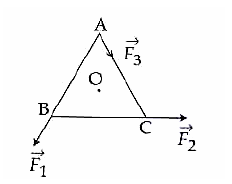A : (F1+F2)/2
B : 2(F1+F2)
C : F1+F2
D : F1-F2

•
.

Solution :
Taking anticlockwise torque
F1d + F2d - F3d = 0 (where d = perpendicular distance of the centre O from each side)
F3 = F1 + F2

•   Question 11

When a mass is rotating in a plane about a fixed point, its angular momentum is directed along

B : the tangent to the orbit
C : a line perpendicular to the plane of rotation
D : the line making an angle of 45° to the plane of rotation.

•
.

Solution :
L=r x mv

•   Question 12

A spherical planet has a mass MP and diameter DP. A particle of mass m falling freely near the surface of this planet will experience an acceleration due to gravity, equal to

A : GMP/ DP2
B : 4GMPm/ DP2
C : 4GMP/ DP2
D : GMPm/ DP2

•
.

Solution :
g = GMP/ RP2
where RP = DP/2

•   Question 13

A geostationary satellite is orbiting the earth at a height of 5R above that surface of the earth, R being the radius of the earth. The time period of another satellite of the earth. The time period of another satellite in hours at a height of 2R from the surface of the earth is

A : 6√2
B : 6/√2
C : 5
D : 10

•
.

Solution :
According to Kepler's third law, T2 ∝ r3
T2/T1 = (r2/r1)3/2
Given T1 = 24 h r1 = R + h1 = R + 5R = 6R
r2 = R + h2 = R + 2R = 3R

On solving we get 6√2

•   Question 14

The height at which the weight of a body becomes 1/16th , its weight on the surface of earth (radius R), is

A : 3R
B : 4R
C : 5R
D : 15R

•
.

Solution :
Wh/W = mg/mg = (R/(R+h))2 = 1/16
h=3R

•   Question 15

Two sources of sound placed close to each other, are emitting progressive waves given by y1 = 4 sin 600 πt and y2 = 5 sin 608 πt: An observer located near these two sources of sound will hear

A : 8 beats per second with intensity ratio 81 : 1 between waxing and waning
B : 4 beats per second with intensity ratio 81 : 1 between waxing and waning
C : 4 beats per second with intensity ratio 25 : 16 between waxing and waning
D : 8 beats per second with intensity ratio 25 : 16 between waxing and waning

•
.

Solution :
Comapare both equations with y = a sin 2πft we get
f1=300Hz
f2=304Hz
Number of beats = f2-f1 = 4s-1
Imax/Imin = ((a1 + a2)/(a1 - a2))
((4+5)/(4-5))2 = 81/1

•   Question 16

When a string is divided into three segments of length l1, l2 and l3, the fundamental frequencies of these three segments are v1, v2 and v3 respectively. The original functamental frequency (v) of the string is :

A : 1/v = 1/v1 + 1/v2 + 1/v3
B : 1/√v = 1/√v1 +1/√v2 +1/√v3
C : √v = √v1 + √v2 + √v3
D : v = v1 +v2 +v3

•
.

Solution :
Length of the string l = l1 + l2 + l3 v ∝ 1/l

•   Question 17

One mole of an ideal gas goes from an initial state A to final state B via two processes : It first undergoes isothermal expansion from volume V to 3V and then its volume is reduced from 3V to V at constant pressure. The correct P-V diagram representing the two processes is:

A :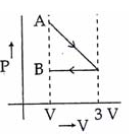B :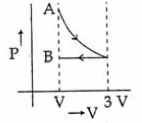C :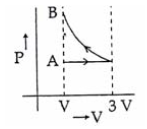D :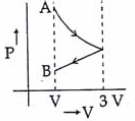•
.

Solution :
For isothermal expansion P-V curve is rectangular hyperbola (clockwise curve)

•   Question 18

A thermodynamics system is taken trough the cycle ABCD as shown in figure. Heat rejected by the gas during the cycle is: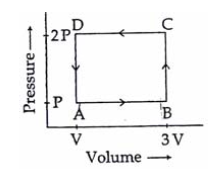A : 1/2 PV
B : PV
C : 2PV
D : 4PV

•
.

Solution :
For given cyclic process, ΔU = 0
Q = W (From first law of thermodynamic Q = ΔU + W) Also W = - area enclosed by the curve = - P * 2V
Heat rejected = 2PV

•   Question 19

Liquid oxygen at 50 K is heated to 300 K at constant pressure of 1 atm. The rate of heating is constant. Which one of the following graphs represents the variation of temperature with time ?

A :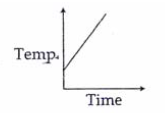B :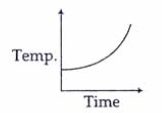C :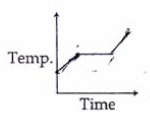D :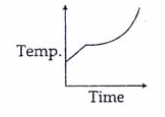•
.

Solution :
At first temperature will increase then there will be state change from liquid to gas.

•   Question 20

If the radius of a star is R and it acts as a black body, what would be the temperature of the star, in which the rate of energy production is Q ?(&sigma is Stefan's constant)

A : (4πR2Q/σ)1/4
B : (Q/4πR2Qσ)1/4
C : Q/4πR2σ
D : (Q/4πR2Qσ)-1/2

•
.

Solution :
Using Stefan's law, the rate of energy production is Q = E * A

•   Question 21

A coil of resistance 400Ω is placed in a magnetic filed. if the magnetic flux φ (wb) linked with the coil varies with times t(sec) as
φ = 50t2 + 4
The current is the coil at t = 2 sec is

A : 2A
B : 1A
C : 0.5A
D : 0.1A

•
.

Solution :
i = |(-dφ/dt)/R| = 0.5A

•   Question 22

The current (I) in the inductance is varying with time according to the plot shown in figure.
Which one of the following is the correct variation of voltage with time in the coil ?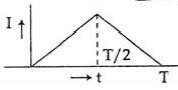A :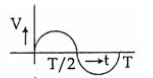B :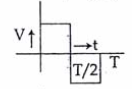C :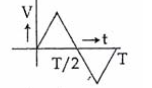D :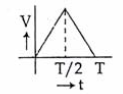•
.

Solution :
|V|=|-Ldi/dt| therefore answer will be best represented by graph -2

•   Question 23

In an electrical circuit R, L, C and an a.c. voltage source are all connected in series. When L is removed from the circuit, the phase difference between the voltage and the current in the ciruit is π/3 . If instead, C is removed from the circuit, the phase difference is again π/3 . The power factor of the circuit is

A : 1
B : √3/2
C : 1/2
D : 1/√2

•
.

Solution :
It is the condition of resonance therefore phase difference between voltage and current is zero and power factor cos &phi = 1

•   Question 24

A ring is made of a wire having a resistance R0 = 12Ω. Find the points A and B, as shown in the figure, at which a current carrying conductor should be connected so that the resistance R of the sub circuit between these points is equal to 8/3Ω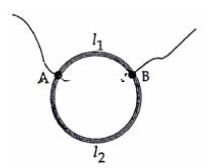A : l1/l2 = 3/8
B : l1/l2 = 1/2
C : l1/l2 = 5/8
D : l1/l2 = 1/3

•
.

Solution :
Since R∝l
According to problem R1 + R2 = 12Ω and (R1 * R2)/(R1 + R2) = 8/3Ω
On solving this we get R1 = 4Ω and R2 = 8Ω therefore the ratio l1/l2 =1/2

•   Question 25

If voltage across a bulb rated 220 Volts. 100 Watt drops by 2.5% of its rated value the percentage of the rated value by which the power would decrease is

A : 5%
B : 10%
C : 20%
D : 2.5%

•
.

Solution :
Power P = V2/R
For small variation,ΔP/P * 100% = 2*ΔV/V * 100%
therefore power would decrease by 5%

•   Question 26

In the circuit shown the cells A and B have negligible resistance. For VA = 12V, R1 = 500Ω and R = 100Ω the galvanometer (G) shows no deflection. The value of VB is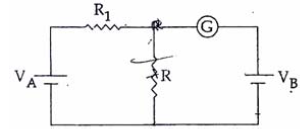A : 12V
B : 6V
C : 4V
D : 2V

•
.

Solution :
By using kirchoff voltage law the potential difference across R is 2 volt

•   Question 27

The electric field associated with an e. m. wave in vacuum is given by E = î 40 cos (kz � 6 � 108t) , where E, z and t are in volt/m, meter and seconds respectively. The value of wave vector k is :

A : 6 m-1
B : 3 m-1
C : 2 m-1
D : 0.5 m-1

•
.

Solution :
Equation of Electromagnetic wave is E = E0 cos (kz - ω t) and speed of EM wave V = ω/k
By comparing k = ω/v = 6 * 10 8/3* 10 8

•   Question 28

What is the flux through a cube of side 'a' if a point charge of q is at one of its corner

A : q/ε0
B : (q/2ε0) * 6a2
C : 2q/ε0
D : q/8ε0

•
.

•   Question 29

An electric dipole of moment 'p' is placed in an electric field of intensity 'E'. The dipole acquires a position such that the axis of the dipole makes an angle θ with the direction of the field. Assuming that the potential energy of the dipole to be zero when θ = 90°, the torque and the potential energy of the dipole will respectively be

A : pEsinθ , 2pEcosθ
B : pEcosθ,-pEsinθ
C : pEsinθ,-pEcosθ
D : pEsinθ,-2pEcosθ

•
.

Solution :
Toque = PEsinθ and Potential energy U = -PEcosθ

•   Question 30

Four point charge -Q, -q, 2q and 2Q are placed, one at each corner of the square. The relation between Q and q for which the potential at the centre of the square is zero is

A : Q = q
B : Q = 1/q
C : Q = -q
D : Q = -1/q

•
.

Solution :
Potential V at centre of the square =
-kQ/r - kq/r + k2Q/r + k2q/r = 0
So Q = -q

•   Question 31

A compass needle which is allowed to move in a horizontal plane is taken to a geomagnetic pole. It.

A : Will stay in north-south direction only
B : Will stay in east-west direction only
C : Will becomes rigid showing no movement
D : Will stay in any position

•
.

Solution :
Will remain in any position at geomagnetic north and south pole

•   Question 32

A milli voltmeter of 25 milli volt range is to be converted into an ammeter of 25 ampere rage. The value (in ohm) of necessary shunt will be

A : 1
B : 0.05
C : 0.001
D : 0.01

•
.

Solution :
If the resistance of the meter is G then the full scale deflection current ig = 25mV/G ampere

The Value of shunt required for converting it into ammeter of range 25 ampere is S = igG/i-ig
so 25mV/25

•   Question 33

Two similar coils of radius R are lying concentrically with their planes at right angles to each other. The currents flowing in them are I and 2I, respectively. The resultant magnetic field induction at the centre will be

A : µ0I/2R
B : µ0I/R
C : √5µ0I/2R
D : 0I/2R

•
.

Solution :
Magnetic field at the centre of circular current carrying coil of radius R and current I is =
µ0I/2R = B
Magnetic field at the centre of circular current carrying coil of radius R and current 2I is =
µ02I/2R = 2B
The resulting magnetic field will be =
√(B2+4B2)=√5B

•   Question 34

An alternating electric field, of frequency v, is applied across the dees (radius = R) of a cyclotron that is being used to accelerated protons (mass = m). The operating magnetic field (B) used in the cyclotron and the kinetic energy (K) of the proton beam, produced by it, are given by

A : B = 2πmv/e and K = 2mπ2v2R2
B : B = mv/e and K = m2πvR2
C : B = mv/e and K = 2mπ2v2R
D : B = 2πmv/e and K = m2πvR2

•
.

Solution :
frequency v = eB/2πm so B = 2πmv/e
Kinetic energy K = 1/2 mv2

•   Question 35

The magnifying power of a teloscope is 9. When it is adjusted for parallel rays the distance between the objective and eyepiece is 20 cm. The focal length of lenses are

A : 18 cm, 2 cm
B : 11 cm, 9 cm
C : 10 cm, 10 cm
D : 15 cm, 5 cm

•
.

Solution :
L = fo + fe = 20cm
M = fo/fe = 9

•   Question 36

A ray of light is incident at an angle of incidence, i, on one face of a prism of angle A (assumed to be small) and emerges normally from the opposite face. It the refractive index of the prism is µ, the angle of incidence i, is nearly equal to

A : A/µ
B : A/2µ
C : µA
D : µA/2

•
.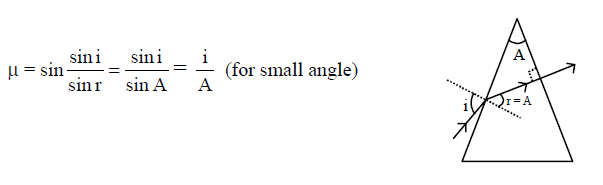•   Question 37

A concave mirror of focal length 'f1' is placed at a distance of 'd' from a convex lens of focal length 'f2' A beam of light coming from infinity and falling on this convex lens- concave mirror combination returns to infinity. The dsitance 'd' must equal

A : 2f1 + f2
B : -2f1 + f2
C : f1 + f2
D : -f1 + f2

•
.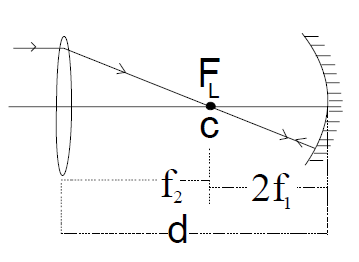•   Question 38

When a biconves lens of glass having refractive index 1.47 is dipped in a liqud, it acts as a plane sheet of glass. This implies that the liquid must have refractive index

A : greater than that of glass
B : less than that of glass
C : equal to that of glass
D : less than one

•
.

Solution :
1/f = (µ21 - 1)(1/R1 - 1/R2)
For power = 1/f = 0,
µ2 = µ1

•   Question 39

An α-particle moves in a circular path of radius 0.83 cm in the presence of a magnetic field of 0.25 Wb/m2. The de-Broglie wavelength associated with the particle will be

A : 10Å
B : 0.01Å
C :
D : 0.1Å

•
.

Solution :
radius R = mv/qB and de- Broglie wavelength λ = h/mv on solving we get λ= 0.01Å

•   Question 40

Monochromatic radiation emitted when electron on hydrogen atom jumps from first excited to the ground state irradiates a photosensitive material. The stopping potential is measured to be 3.57 V. The threshold frequency of the material is

A : 1.6 * 1015 Hz
B : 2.5 * 1015 Hz
C : 4 * 1015 Hz
D : 5 * 1015 Hz

•
.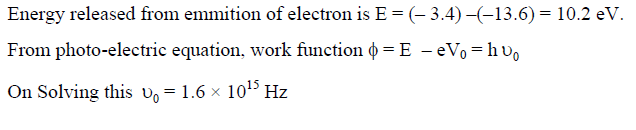•   Question 41

A 200 W sodium street lamp emits yellow light of wavelength 0.6 µm. Assuming it to be 25% efficient in converting electrical energy to light, the number of photons of yellow light it emits per second is

A : 62 * 1020
B : 3 * 1019
C : 1.5 * 1020
D : 6 * 1018

•
.

Solution :
Efficient power P = N/t * hc/λ = 200*0.25
On Solving N/t = 50* λ/hc

•   Question 42

Electron in hydrogen atom first jumps from third excited state to second excited state and then from second excited to the first excited state. The ratio of the wavelengths λ1 : λ2 emitted in the two cases is

A : 27/5
B : 20/7
C : 7/5
D : 27/20

•
.

Solution :
Wavelength observed from transition of ni to nf is
1/[1/nf2 - 1/ni2]
For λ1 , ni = 4, nf = 3 For λ2 ,ni = 3, nf = 2

•   Question 43

An electron of a stationary hydrogen atom passes from the fifth energy level to the ground level. The velocity that the atom acquired as a result of photon emission will be

A : 25m/24hR
B : 24m/24hR
C : 24m/25hR
D : 25m/24hR

•
.

Solution :
v = h/λm

•   Question 44

If the nuclear radius of 27Al is 3.6 Fermi, the approximate nuclear radius of 64Cu in Fermi is

A : 4.8
B : 3.6
C : 2.4
D : 1.2

•
.

Solution :
Nuclear radius R ∝ A1/3 where A is mass number

•   Question 45

A mixture consists of two radioactive materials A1 and A2 with half lives of 20s and 10s respectively. Initially the mixture has 40g of A1 and 160 g of A2. The amount of the two in the mixture will become equal after :

A : 20s
B : 40s
C : 60s
D : 80s

•
.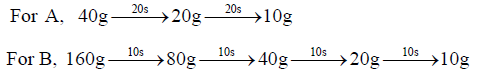•   Question 46

Two ideal diodes are connected to a battery as shown in the circuit. The current supplied by the battery is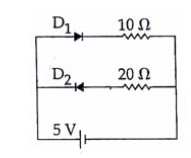A : 0.25 A
B : 0.5 A
C : 0. 75 A
D : Zero

•
.

Solution :
Current will flow only in diode D1 therefore current supplied by the battery is 0.5 A Since D1 is forward bias and D2 is reverse bias.

•   Question 47

The figure shown a logic circuit with two inputs A and B and the output C. The voltage wave farms across A, B and C are given. The logic circuit gate is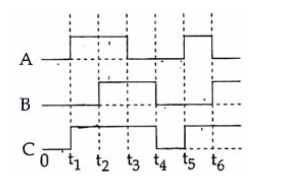A : AND gate
B : NAND gate
C : OR gate
D : NOR gate

•
.

Solution :
The Logic of the OR gate is if any of the input is one then output is one

•   Question 48

In a CE transistor amplifier, the audio singal voltage across the collector resistance of 2kΩ is 2V. If the v base resistance is 1 kΩ and the current amplification of the transistor is 100, the input signal voltage is

A : 1 mV
B : 10 mV
C : 0.1 V
D : 1.0 V

•
.

Solution :
Voltage amplification Vo/Vi = current gain * Ro/Ri

•   Question 49

C and Si both have same lattice structure, having 4 bonding electrons in each. However, C is insulator where as Si is intrinsic semiconductor. This is because

A : The four bonding electrons in the case of C lie in the second orbit, whereas in the case of Si they lie in the third.
B : The four bonding electrons in the case of C lie in the third orbit, whereas for Si they lie in the fourth orbit.
C : In case of C the valence band is not completely filled at absolute zero temperature
D : In case of C the conduction bans is partly filled even at absolute zero temperature

•
.

Solution :
The four bonding electrons in the case of C lie in the second orbit, whereas in the case of Si they lie in the third.

•   Question 50

Transfer characteristics [output voltage (V0) vs input voltage (Vi)] for a base biased transistor in CE configuration is as show in the figure. For using transistor as a switch, it is used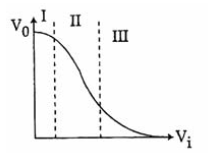A : in region II
B : in region I
C : in region III
D : both in region (I) and (III)

•
.

Solution :
For using transistor as a switch, it is used in cut off state and saturation state only.

•   Question 51

In a zero-order reaction for every 10° rise of temperature, the rate is doubled. If the temperature is increased from 10°C to 100°C, the rate of the reaction will become:

A : 64 times
B : 128 times
C : 256 times
D : 512 times

•
.

Solution :
29 = 512

•   Question 52

Which one of the following pairs is isostructural (i.e. having the same shape and hybridization) ?

A : [NF3 and BF3]
B : [BF4- and NH4+]
C : [BCl3 and BrCl3]
D : [NH3 and NO3-]

•
.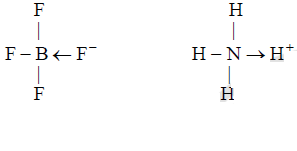•   Question 53

In which of the following reactions, standard reaction entropy change (ΔS°) is positive and standard gibb's energy change (ΔG°) decreases sharply with increasing temperature ?

A : Mg(s) + 1/2O2(g) → MgO(s)
B : 1/2C graphite + 1/2O2(g) → 1/2 CO2
C : C graphite + 1/2O2(g) → CO(g)
D : CO(g) + 1/2O2(g) → CO2

•
.

Solution :
ΔS = +ve
ΔG = ΔH - TΔS, ΔH=-ve
ΔG = +ve

•   Question 54

In a reaction, A + B → Product , rate is doubled when the concentration of B is doubled, and rate increases by a factor of 8 when the concentrations of both the reactants (A and B) are doubled, rate law for the reaction can be written as

A : Rate = k[A][B]
B : Rate = k[A]2[B]
C : Rate = k[A][B]2
D : Rate = k[A]2[B]2

•
.

Solution :
r = K[A]m[B]n
wrt B r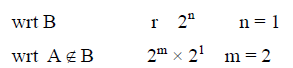•   Question 55

Limiting molar conductivity of NH4OH (i.e.Δ°m (NH4OH)) is equal to

A : Δ°m(NH4OH) + Δ°m(NH4Cl) - Δ°m(HCl)
B : Δ°m(NH4Cl)+ Δ°m(NaOH)- Δ°m(NaCl)
C : Δ°m(NH4Cl)+ Δ°m(NaCl) - Δ°m(NaOH)
D : Δ°m (NaOH) + Δ°m (NaCl) - Δ°m (NH4Cl)

•
.

Solution :
NH4Cl + NaOH → NaCl + NH4OH

•   Question 56

Which of the following species contains three bond pairs and one lone pair around the central atoms ?

A : NH-2
B : PCl3
C : H2O
D : BF3

•
.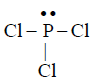•   Question 57

Buffer solutions have constant acidity and alkalinity because

A : They have large excess of H+ or OH- ions
B : They have fixed value of pH
C : These give unionised acid or base on reaction with added acid or alkali
D : Acids and alkalies in these solutions are shielded from attack by other ions

•
.

•   Question 58

In Freundlich Adsorption isotherm, the value of 1/n is :

A : 1 in case of physical adsorption
B : 1 in case of chemisorption
C : between 0 and 1 in all cases
D : between 2 and 4 in all cases

•
.

•   Question 59

pH of a saturated solution of Ba(OH)2 is 12. The value of solubility product (KSP) of Ba(OH)2 is

A : 4.0 * 10-6
B : 5.0 * 10-6
C : 3.3 * 10-7
D : 5.0 * 10-7

•
.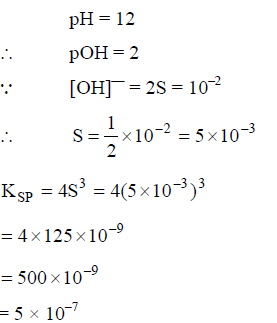•   Question 60

When Cl2 gas reacts with hot and concentrated sodium hydroxide solution, the oxidation number of chlorine changes from :

A : Zero to -1 and zero to +3
B : Zero to +1 and zero to -3
C : Zero to +1 and zero to -5
D : Zero to -1 and zero to +5

•
.

Solution :
Cl2 + NaOH (Hotconc.)→NaCl +NaClO3

•   Question 61

Which one of the following statements is incorrect about enzyme catalysis ?

A : Enzymes are denaturated by ultraviolet rays and at high temperature
B : Enzymes are least reactive at optimum temperature
C : Enzymes are mostly proteinous in nature
D : Enzyme action is specific

•
.

•   Question 62

pA and pB are the vapour pressure of pure liquid components, A and B, respectively of an ideal binary solution. If xA represents the mole fraction of component A, the total pressure of the solution will be:

A : pB + xA(pB - pA)
B : pB + xA(pA - pB)
C : pA + xA(pB - pA)
D : pA + xA(pA - pB)

•
.

Solution :
P = pAxA + pBxB
P = pA * XA + pB(1-xA)
P = pB + xA(pA - pB)

•   Question 63

The protecting power of lyophilic colloidal sol is expressed in term of

A : Critical miscelle concentration
B : Oxidation number
C : Coagulation value
D : Gold number

•
.

•   Question 64

Maximum number of electrons in a subshell with l = 3 and n = 4 is :

A : 10
B : 12
C : 14
D : 16

•
.

Solution :
Subshell 4f = election = 14

•   Question 65

50 mL of each gas A and of gas B takes 150 and 200 seconds respectively for effusing through a pin hole under the similar conditions. If molecular mass of gas B is 36, the molecular mass of gas A will be ?

•
.

Solution :

•   Question 66

Standard enthalpy of vapourisation ?vapH? for water at 100°C is 40.66 kJ mol-1. The internal energy of vaporisation of water at 100°C (in kJmol-1) is :
(Assume water vapour to behave like an ideal gas)

A : +43.76
B : +40.66
C : +37.56
D : -43.76

•
.

Solution :
H2O(l) → H2O(g)
ΔH = ΔE + ΔnRT
40.66 = ΔE + 1*8.314*373/1000
ΔE = 37.5kJ

•   Question 67

The number of octahedral void(s) per atom present in a cubic close-packed structure is :

A : 2
B : 4
C : 1
D : 3

•
.

•   Question 68

The correct set of four quantum numbers for the valence electron of rubidium atom (Z = 37) is

A : 5,0,0,1/2
B : 5,1,0,1/2
C : 5,1,1,1/2
D : 6,0,0,1/2

•
.

Solution :
Rb(37) = [kr]5s1
n = 5, l = 0 m = 0, s = +1/2

•   Question 69

A metal crystallizes with a face-centered cubic lattice. The edge of the unit cell is 408 pm. The diameter of the metal atom is

A : 144 pm
B : 204pm
C : 288pm
D : 408pm

•
.

Solution :
For FCC r = a/2√2
So diameter = a/√2
408/1.414 = 288.5pm

•   Question 70

The enthalpy of fusion of water is 1.435 kcal/mol. The molar entropy change for the melting of ice at 0°C is :

A : 5.260cal /(molK)
B : 0.5260cal /(molK)
C : 10.52 cal /(molK)
D : 21.04 cal /(molK)

•
.

Solution :
ΔS = ΔH/T
=1.435 *1000/273
=5.26

•   Question 71

In which of the following compounds, nitrogen exhibits highest oxidation state ?

A : N3H
B : NH2OH
C : N2H4
D : NH3

•
.

Solution :
Oxidation number of nitrogen
-N3H = -1/3
NH2OH = -1
N2H4 = -2
NH3 = -3

•   Question 72

Aluminium is extracted from alumina (Al2O3) by electrolysis of a molten mixture of :

A : Al2O3 + Na3AlF6 + CaF2
B : Al2O3 + KF+ Na3AlF6
C : Al2O3 + HF + NaAlF4
D : Al2O3 + NaAlF4 + CaF2

•
.

•   Question 73

Which of the statements is not true ?

A : K2Cr2O7 solution in acidic medium is orange
B : K2Cr2O7 solution becomes yellow on increasing the pH beyond 7
C : On passing H2S through acidified K2Cr2O7 solution, a milky colour is observed
D : Na2Cr2O7 is preferred over K2Cr2O7 in volumetric analysis

•
.

Solution :
Na2Cr2O7 is hygroscopic

•   Question 74

A mixture of potassium chlorate, oxalic acid and sulphuric acid is heated. During the reaction which element undergoes maximum change in the oxidation number ?

A : Cl
B : C
C : S
D : H

•
.

Solution :
Maximum change in oxidation number = Cl

•   Question 75

Which one of the following is an outer orbital complex and exhibits paramagnetic behaviour :

A : [Cr(NH3)6]3+
B : [Co(NH3)6]3+
C : [Ni(NH3)6]2+
D : [Zn(NH3)6]2+

•
.

Solution :
Weak ligand with two unpaired electron

•   Question 76

The ease of adsorption of the hydrated alkali metal ions on an ion-exchange resins follows the order :

A : K+ < Na+ < Rb+ < Li+
B : Na+ < Li+ < K+ < Rb+
C : Li+ < K+ < Na+ < Rb+
D : Rb+ < K+ < Na+ < Li+

•
.

•   Question 77

Equimolar solutions of the following substances were prepared separately. Which one of these will record the highest pH value ?:

A : LiCl
B : BeCl2
C : BaCl2
D : AlCl3

•
.

Solution :
BeCl2 → Ba(OH)2 + HCl
Ba(OH)2 is a strong base

•   Question 78

Sulphur trioxide can be obtained by which of the following reaction :

A : S + H2SO4 Δ→
B : H2SO4 + PCl Δ→
C : C + CaSO2 Δ→
D : Fe2 + (SO4)3 Δ→

•
.

Solution :
Fe2(SO4)3 Δ→ Fe2O3 + 3SO3

•   Question 79

In the extraction of copper from its sulphide ore, the metal is finally obtained by the reduction of cuprous oxide with :

A : Iron sulphide (FeS)
B : Carbon monoxide (CO)
C : Copper (I) sulphide (Cu2S)
D : Sulphur dioxide (SO2)

•
.

Solution :
2Cu2O + Cu2S → 6Cu +SO2

•   Question 80

Identity the wrong statement in the following

A : Atomic radius of the elements increases as one moves down the first group of the periodic table
B : Atomic radius of the elements decreases as one moves across from left to right in then 2nd period of the periodic table
C : Amongst isoelectronic species, smaller the positive charge on the cation, smaller is the ionic radius
D : Amongst isoelectronic species, greater the negative charge on the anion, large is the ionic radius

•
.

Solution :
Ionic size ∝ 1/charge on cation

•   Question 81

Which of the following statements is not valid for oxoacids of phosphorus ?

A : All oxoacids contain tetrahedral four coordinated phosphorous
B : All oxoacids contain atleast one P = O units and one P - OH group
C : Orthophosphoric acid is used in the manufacture of triple superphosphate
D : Hypophosphorous acid is a diprotic acid

•
.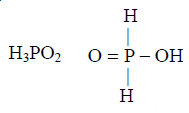•   Question 82

Identify the alloy containing a non-metal as a constituent in it

A : Bell metal
B : Bronze
C : Invar
D : Steel

•
.

Solution :
Steel → Fe + C

•   Question 83

The pair of species with the same bond order is

A : NO, CO
B : N2, O2
C : O2-2, B2
D : O2+,NO+

•
.

Solution :
Both have one

•   Question 84

Bond order of 1.5 is shown by:

A : O22-
B : O2
C : O2+
D : O2-

•
.

Solution :
O2- B.O =1.5

•   Question 85

Which one of the following is a mineral of iron ?

A : Pyrolusite
B : Magnetite
C : Malachite
D : Cassiterite

•
.

Solution :
Fe2O3

•   Question 86

Which one of the alkali metals, forms only, the normal oxide, M2O on heating in air ?

A : Li
B : Na
C : Rb
D : K

•
.

Solution :
4Li + O2 → 2Li2O

•   Question 87

The correct order of decreasing acid strength of trichloroacetic acid (A), trifluoroacetic acid (B), acetic acid (C) and formic acid (D) is :

A : A>B>C>D
B : A>C>B>D
C : B>A>D>C
D : B>D>C>A

•
.

Solution :
Acidicstrength due to I-effect

•   Question 88

In the following reaction :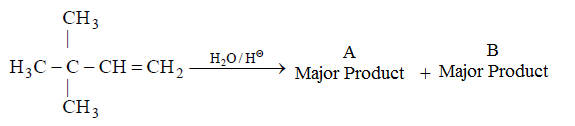A :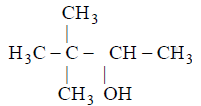B :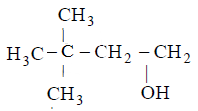C :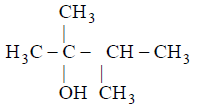D :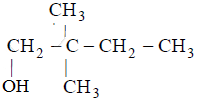•
.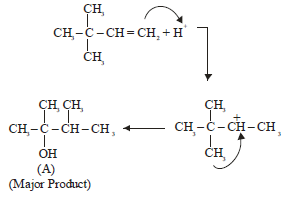•   Question 89

Which nomenclature is not according to IUPAC system ?.

A :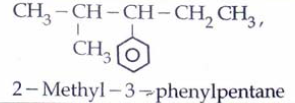B :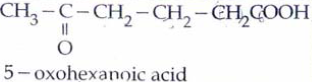C :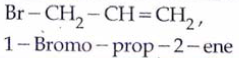D :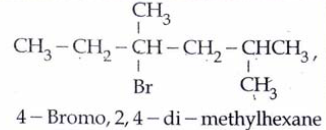•
.

Solution :
Correct Name - 3 Bromo propene

•   Question 90

Among the following compounds the one that is most reactive towards electrophilic nitration is :

A : Toluene
B : Benzene
C : Benzoic acid
D : Nitrobenzene

•
.

Solution :
Due to +R effect of methyl group A) is more reactive towards ESR (Nitration).

•   Question 91

Deficiency of vitamin B1 causes the disease

A : Cheilosis
B : Sterility
C : Convulsions
D : Beri-Beri

•
.

Solution :
Deficiency of B1 cause Beri-Beri.

•   Question 92

Which one of the following sets of monosaccharides forms sucrose ?

A : α-D-Glucopyranose and β-D-fructofuranose
B : β-D-Glucopyranose and α-D-fructofuranose
C : α-D-Glucopyranose and α-D-fructofuranose
D : α-D-Glucopyranose and β-D-fructofuranose

•
.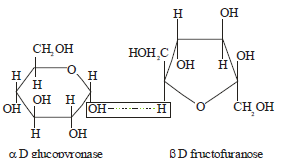•   Question 93

Which one of the following statements regarding photochemical smog is not correct ?:

A : Photochemical smog is formed through photochemical reaction involving solar energy
B : Photochemical smog does not cause irritation in eyes and throat
C : Carbon monoxide does not play any role in photochemical smog formation
D : Photochemical smog is an oxidising agent in character

•
.

Solution :
Photo chemical smog causes irritation in eyes & throat

•   Question 94

In the following sequence of reactions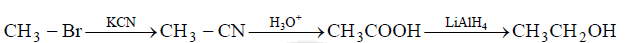A : Acetaldehyde
B : Ethyl alcohol
C : Acetone
D : Methane

•
.•   Question 95

Which one of the following is not a condensation polymer ?

A : Dacron
B : Neoprene
C : Melamine
D : Glyptal

•
.

•   Question 96

Predict the products in the given reaction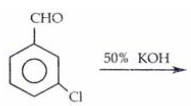A :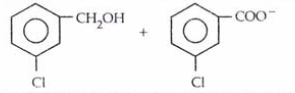B :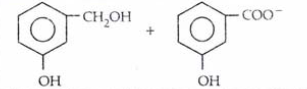C :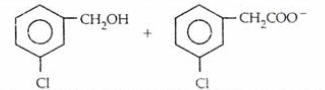D :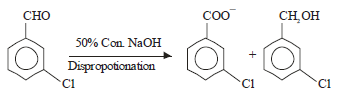•
.

Solution :
Cannizaro reaction•   Question 97

Which of the following acids does not exhibit optical isomerism ?

A : Lacetic acid
B : Tartaric acid
C : Maleic acid
D : α-amino acids

•
.

Solution :
HOOC-CH = CH - COOH (Maleic acid) almost show optical isomerism

•   Question 98

CH3CHO and C6H5CH2CHO can be distinguised chemically by :

A : Tollen's reagent test
B : Fehling solution test
C : Benedict test
D : Iodoform test

•
.

Solution :
Iodoform test
+ve for CH3CHO
-ve for C6H5CH2CHO

•   Question 99

Which of the following statements is false ?

A : The repeat unit in natural rubber is isoprene
B : Both starch and cellulose are polymers of glucose
C : Artificial silk is derived from cellulose
D : Nylon-66 is an example of elastomer

•
.

Solution :
Nylon 6, 6 is a fibre not an elastomer

•   Question 100

Acetone is treated with excess of ethanol in the presence of hydrochloric acid. The product obtained is

A :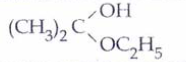B :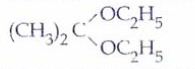C :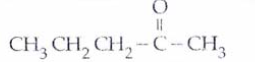D :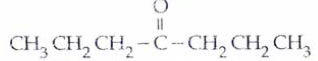•
.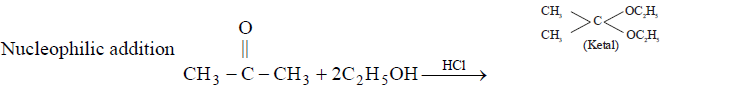•   Question 101

Cycas and adiantum resemble each other in having

A : Cambium
B : Vessels
C : Seeds
D : Motile sperms

•
.

•   Question 102

Gymnosperms are also called soft wood spermatophytes because they lack

A : Thick - walled tracheids
B : Xylem fibres
C : Cambium
D : Phloem fibres

•
.

•   Question 103

Maximum nutritional diversity is found in the group

A : Monera
B : Plantae
C : Fungi
D : Animalia

•
.

•   Question 104

Which one of the following is common to multicellular fungi, filamentous algae and protonema of mosses

A : Mode of Nutrition
B : Multiplication by fragmentation
C : Diplontic life cycle
D : Members of kingdom plantae

•
.

•   Question 105

Which statement is wrong for viruses

A : They have ability to synthesiz nucleic acids and proteins
B : Antibiotics have no effect on them
C : All are parasites
D : All of them have helical symmetry

•
.

•   Question 106

Which one of the following is correct statement

A : Antheridiophores and archegoniophores are present in pteridophytes
B : Origin of seed habit can be traced in pteridophytes
C : Pteridophyte gametophyte has a protonemal and leafy stage
D : In gymnosperm female gametophyte is free living

•
.

•   Question 107

Nuclear membrane is absent in

A : Volvox
B : Nostoc
C : Penicillium
D : Agaricus

•
.

•   Question 108

During gamete formation, the enzyme recombinase participates during

A : Prophase - I
B : Prophase - II
C : Metaphase - I
D : Anaphase - II

•
.

•   Question 109

Which one of the following does not differ in E.coli and Chlamydomonas

A : Cell wall
B : Cell membrane
C : Ribosomes
D : Chromosomal Organization

•
.

•   Question 110

PCR and Restriction Fragment Length Polymorphism are the methods for

A : DNA sequencing
B : Genetic Fingerprinting
C : Study of enzymes
D : Genetic transformation

•
.

•   Question 111

Removal of RNA polymerase -III from nucleoplasm will affect the synthesis of

A : mRNA
B : rRNA
C : tRNA
D : hnRNA

•
.

•   Question 112

Evolution of different species in a given area starting from a point and spreading to other geographical areas is known as

A : Migration
B : divergent evolution
D : Natural selection

•
.

•   Question 113

Removal of introns and joining of exons in a defined order during transcription is called

A : Slicing
B : Splicing
C : Looping
D : Inducing

•
.

•   Question 114

Which one of the following is not a part of a transcription unit in DNA

A : A promoter
B : The structural gene
C : the inducer
D : A terminator

•
.

•   Question 115

An organic substance that can withstand environmental extremes and cannot be degraded by any enzyme is

A : Lignin
B : Cellulose
C : Cuticle
D : Sporopollenin

•
.

•   Question 116

Best defined function of manganese in green plants is

A : Nitrogen fixation
B : Water absorption
C : Photolysis of water
D : Calvin cycle

•
.

•   Question 117

Water containing cavities in vascular bundles are found in

A : Cycas
B : Pinus
C : Sunflower
D : Maize

•
.

•   Question 118

Closed vascular bundles lack

A : Cambium
B : Pith
C : Ground tissue
D : Conjunctive tissue

•
.

•   Question 119

Placentation in Tomato and lemon is

A : Marginal
B : Axile
C : Parietal
D : Free central

•
.

•   Question 120

Companion cells are closely associated with

A : Trichomes
B : Guard cells
C : Sieve elements
D : Vessel elements

•
.

•   Question 121

Vexillary aestivation is characteristic of the family

A : Solanaceae
B : Brassicaceae
C : Fabaceae
D : Asteraceae

•
.

•   Question 122

Phyllode is present in

A : Australian Acacia
B : Opuntia
C : Asparagus
D : Euphorbia

•
.

•   Question 123

The common bottle cork is a product of

A : Xylem
B : Vascular Cambium
C : Dermatogen
D : Phellogen

•
.

•   Question 124

Which one of the following is wrong statement

A : Phosphorus is a constituent of cell membranes, certain nucleic acids and all proteins
B : Nitrosomonas and Nitrobacter are chemoautotrophs
C : Anabaena and Nostoc are capable of fixing nitrogen in free- living state also
D : Root nodule forming nitrogen fixers live as aerobes under free living conditions

•
.

•   Question 125

How many plants in the list give below have composite fruits that develop from an inflorescence Walnut, poppy, radish, fig, pineapple, apple, tomato, mulberry

A : 2
B : 3
C : 4
D : 5

•
.

•   Question 126

Cymose inflorescence is present in

A : Trifolium
B : Brassica
C : Solanum
D : Sesbania

•
.

•   Question 127

Which one of the following is correctly matched

A : Potassium - Readily immobilisation
B : Bakane of rice seedlings - F skoog
C : Passive transport of nutrients - ATP
D : Apoplast - Plasmodesmata

•
.

•   Question 128

A process that makes important difference between C3 and C4 plants is

A : Photosynthesis
B : Photorespiration
C : Transpiration
D : Glycolysis

•
.

•   Question 129

The correct sequence of cell organelles during photorespiration is

A : Chloroplast, mitochondria, peroxisome
B : Chloroplast, - vacuole - peroxisome
C : Chloroplast, - Golgiboidies - mitochondria
D : Chloroplast, Rough Endoplasmic reticulum- Dictyosomes

•
.

•   Question 130

The coconut water and the edible part of coconut are equivalent to

A : Mesocarp
B : Embryo
C : Endosperm
D : Endocarp

•
.

•   Question 131

The gynoecium consists of many free pistils in flowers of

A : Papaver
B : Michelia
C : Aloe
D : Tomato

•
.

•   Question 132

Which one of the following is correctly matched

A : Chlamydomonas - Conidia
B : yeast - Zoospores
C : Onion - Bulb
D : Ginger - Sucker

•
.

•   Question 133

Both, autogamy and geitonogamy are prevented in

A : Castor
B : Maize
C : Papaya
D : Cucumber

•
.

•   Question 134

Even in absence of pollinating agents seed seting is assured in

A : Salvia
B : Fig
C : Commellina
D : Zostera

•
.

•   Question 135

Which one of the following areas in India, is a hotspot of biodiversity

A : Sunderbans
B : Western Ghats
C : Eastern Ghats
D : Gangetic plain

•
.

•   Question 136

Which one of the following is not a functional unit of an ecosystem

A : Productivity
B : Stratification
C : Energy flow
D : Decomposition

•
.

•   Question 137

The upright pyramid of number is absent in

A : Lake
B : Grassland
C : Pond
D : Forest

•
.

•   Question 138

Which one of the following is not a gaseous biogeochemical cycle in ecosystem

A : Nitrogen Cycle
B : Carbon Cycle
C : Sulphur Cycle
D : Phosphorus Cycle

•
.

•   Question 139

Which one of the following is a wrong statement

A : Greenhouse effect is a natural Phenomenon
B : Eutrophication is a natural phenomenon in freshwater bodies
C : Most of the forests have been lost in tropical areas
D : Ozone in upper part of atmosphere is harmful to animals

•
.

•   Question 140

The highest number of species in the world is represented by

A : Algae
B : Lichens
C : Fungi
D : Mosses

•
.

•   Question 141

Yeast is used in the production of

B : Cheese and butter
C : Citric acid and lactic acid
D : Lipase and pectinase

•
.

•   Question 142

Which one of the following microbes forms symbiotic association with plants and helps then in their nutrition

A : Glomus
B : Trichoderma
C : Azotobacter
D : Aspergillus

•
.

•   Question 143

A single strand of nucleic acid tagged with a radioactive molecule is called

A : Plasmid
B : Probe
C : Vector
D : Selectable marker

•
.

•   Question 144

A patient brought to a hospital with myocardial infarction is normally immediately given

A : Cyclosporin - A
B : Statins
C : Penicillin
D : Streptokinase

•
.

•   Question 145

A nitrogen - fixing microbe associated with Azolla in rice fields is

A : Frankia
B : Tolypothrix
C : Spirulina
D : Anabaena

•
.

•   Question 146

Which one is a true statement regarding DNA polymerase used in PCR

A : It is isolated from a virus
B : It remains active at high temperature
C : It is used to ligate introduced DNA in recipient cell
D : It serves as a selectable marker

•
.

•   Question 147

Consumption of which one of the following foods can prevent the kind of blindness associated with vitamin 'A' deficiency

A : golden rice
B : Bt- Brinjal
C : Flaver Savr Tomato
D : Canolla

•
.

•   Question 148

Which one of the following is a case of wrong matching

A : Micropropagation - In vitro production of plants in large numbers
B : Callus - Unorganised mass of cells produced in tissue culture
C : Somatic hybridization - Fusion of two diverse cells
D : Vector DNA - site for tRNA synthesis

•
.

•   Question 149

Which part would be most suitable for raising virus free plants for microporpagation

A : Meristem
B : Node
C : Bark
D : Vascular tissue

•
.

•   Question 150

For transformation, micro - particles coated with DNA to be bombarded with gene are made up of

A : Silicon or platinum
B : Gold or Tungsten
C : Silver or platinum
D : Platinum or zinc

•
.

•   Question 151

The cyanobacteria are also referred to as

A : Slime moulds
B : Blue green algae
C : Protists
D : Golden algae

•
.

•   Question 152

Which one single organism or the pair of organisms is correctly assigned to its or their named taxonomic group

A : Yeast used in making bread and beer is a fungus
B : Nostoc and Anabaena are examples of protista
C : Paramecium and Plasmodium belong to the same kingdom as that of Penicillium
D : Lichen is composite organism formed from the symbiotic association of an algae and a protozoan

•
.